# Extracting Characters from Text Using Text Formulas

To extract the characters, words, first, middle and last name from a cell, we use the formulas “LEFT”, “RIGHT”, “MID”, “LEN”, and “SEARCH” in Microsoft Excel.

LEFT: Returns the first character(s) in a text string based on the number of characters specified.

Syntax of “LEFT” function:            =LEFT (text,[num_chars])

For Example:We have name in a cell and we want to extract the first word from the cell.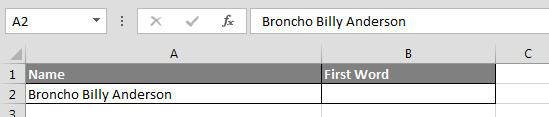To extract the first name from the cell, enter the formula in cell:-

=LEFT (A2, 7), function will return“Broncho”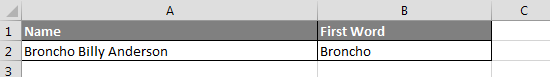RIGHT: Returns the last character(s) in a text string based on the number of characters specified.

Syntax of “RIGHT” function:        =RIGHT (text, [num_chars])

For Example:We have name in a cell and we want to extract the last word from the cell.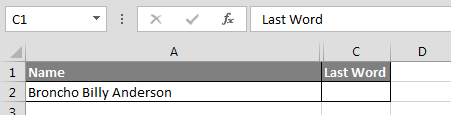To extract the last name from the cell, enter the formula in cell:-

=RIGHT(A2,8), andfunction will return “Anderson”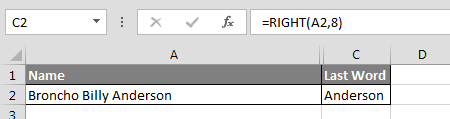MID: Returns a specific number of character(s) from a text string, starting at the position specified based on the number of characters specified.

Syntax of “MID” function:=MID (text,start_num,num_chars)

For Example:We have name in a cell and we want to extract the middle word from the cell.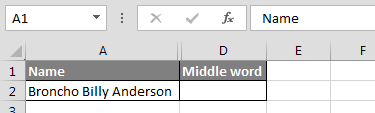To extract the middle name from the cell, enter the formula in cell:-

=MID(A2,9,5), function will return “Billy”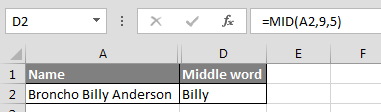LEN: Returns the number of characters in a text string.

Syntax of “LEN” function:             =LEN (text)

For Example: We have name in a cell and we want to calculate the length of text.To calculate the length of text, enter the formula:-

=LEN(A2), function will return22SEARCH:The SEARCH function returns the starting position of a text string which it locates from within the text string.

Syntax of “SEARCH” function:      =SEARCH (find_text,within_text,[start_num])

For Example:We have name in a cell and we want to search “Billy” within text.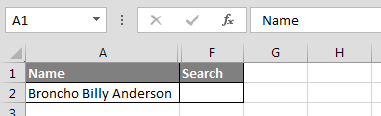To find out the Billy in the cell, enter the formula in cell:-

=SEARCH("Billy",A2,1), function will return 9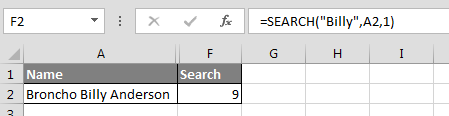These are the formulas which we use to extract characters from text, using text formulas in Microsoft Excel.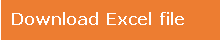Terms and Conditions of use

The applications/code on this site are distributed as is and without warranties or liability. In no event shall the owner of the copyrights, or the authors of the applications/code be liable for any loss of profit, any problems or any damage resulting from the use or evaluation of the applications/code.